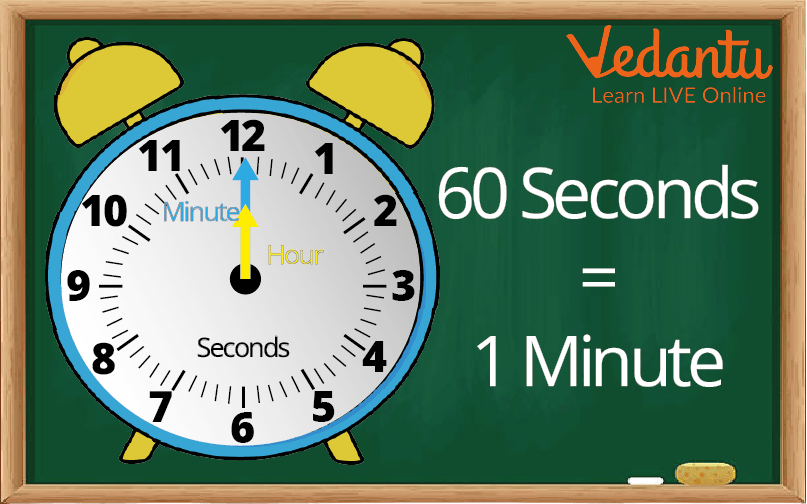Courses
Courses for Kids
Free study material
Offline Centres
More

# How to Convert Minutes into Seconds ?Last updated date: 06th Dec 2023
Total views: 146.7k
Views today: 3.46k## Introduction to Time

Today we will learn about time. As we all know that time is very precious in our life, We should manage time very smartly in our daily life. Time is the invention of sundials. Time is used to measure our daily events and activities. Time has different units and moves in one direction.Time

In a year there are 365 days and in a day there are 24 hours. In 1 hour there are always 60 minutes and in 1 minute there are always 60 seconds. In today’s topic we will learn how many seconds are there in 1 minute and how to convert minutes into seconds.

## What is Time in Minutes?

There are 12 big divisions in a clock and 1 big division is equal to 5 small divisions and each small division is called Minute. In a clock there are three hands: Hour hand, Minute hand and Second hand. The bigger hand is called the minute hand.

## How Many Seconds are There in 1 Minute?

We can understand it by an example: A teacher in a class distributes 60 toffees costing Rs. 60 to all the students and there was a brilliant student who always scored high marks in examinations. The teacher gave him a chocolate which cost Rs. 60.

So we can assume that a brilliant student is minute and the other students are seconds.

The cost of one chocolate is equal to 60 toffees.

So there are 60 seconds in 1 minuteConversion of Time

## How to Convert 1 Minute into Seconds?

• 1 minute is equal to 60 seconds

• 1 minute = 60 seconds

So for converting minutes into seconds we will multiply given minutes by 60.

## For Example:

Q1. Convert 5 minutes into seconds?

Ans:  1 minute = 60 seconds

Then, 5 minutes = (5 × 60) seconds = 300 seconds

Q2. Convert 42 minutes into seconds?

Ans:  1 minute = 60 seconds

Then, 42 minutes = (42 × 60) seconds = 2520 seconds

Q3. Convert 58 minutes into seconds?

Ans:  1 minute = 60 seconds

Then, 58 minutes = (58 × 60) seconds = 3480 seconds

Q4. Convert 6 minutes 55 seconds into seconds?

Ans:  1 minute = 60 seconds

Then, 6 minutes 55 seconds = (6 × 60) seconds + 55 seconds

= 360 seconds + 55 seconds

= 415 seconds

Q5. Convert 36 minutes 18 seconds into seconds?

Ans: 1 minute = 60 seconds

Then, 36 minutes 18 seconds = (36 × 60) seconds + 18 seconds

= 2160 seconds + 18 seconds

= 2178 seconds

Q6. Rohit swims 8 m length of a swimming pool. His target is to swim the length in under 7 minutes. It takes him 440 seconds. Explain why Rohit did not achieve his target ?

Ans: The length of swimming pool is 8m

His target to swim is 7 minutes = (7 × 60) second = 420 seconds

But he takes 440 seconds  = 7 minutes and 20 seconds

He did not complete his target because 440 seconds is more than 420 seconds.

Q1. Convert 48 minutes into seconds?

Ans: 2880 seconds

Q2. Convert 25 minutes 54 seconds into seconds?

Ans: 1554 seconds

Q3. Reena Swings 18m length of a swimming pool. His target is to swim the length in under 20 minutes. It takes him 1245 seconds. Explain why Reena did not achieve his target ?

Ans: 1245 seconds is more than 1200 seconds

## Summary

There are 365 days in a year and 24 hours in a day. There are always 60 minutes in an hour and 60 seconds in a minute.

A clock has 12 main divisions, each of which is divided into 5 smaller divisions, each of which is referred to as a minute. The hour hand, minute hand, and second hand are the three hands on a clock. The minute hand refers to the larger hand.

One hour has 60 minutes, and one minute has 60 seconds.

## FAQs on How to Convert Minutes into Seconds ?

1. Every 3 minutes a clock adds 5 seconds. If the clock began working at 7 a.m., what time would it be in the incorrect clock at 4 p.m. on the same day?

A clock adds 5 seconds every 3 minutes, therefore it gains 50 seconds in 30 minutes and 100 seconds in 60 minutes. That is, it will gain 100 seconds in one hour. Since the clock started at 7 a.m and the current time is 4 p.m the clock has worked for a total of 9 hours. We know that it adds 100 seconds in one hour and 900 seconds in nine hours. The result of converting 900 seconds to minutes is 15 minutes. As the clock gains, this increment shows that it is 15 minutes quicker.

2. There are two wall clocks in a house, one in the kitchen and other in the bedroom. Right now, the time displayed on both watches is 12 a.m. The clock in the bedroom increases by five minutes each hour, while the clock in the kitchen increases by five minutes per hour. When will both watches display the same time?

The faster clock completes one hour in 5 minutes.The slower clock moves 5 minutes slower every hour.

As a result, the faster clock will trace 5+5=10 minutes more in 1 hour than the slower clock.When compared to the slower clock, the faster clock will record 106=60 minutes (an hour) more in 6 hours.The faster clock will trace one hour more than the slower clock in 612=72 hours since the faster clock determines 12 hours more than the slower clock. Both clocks will reflect the same time at this point.  That is  after exactly  72 hours  both clocks will display the same time (or 3 days).